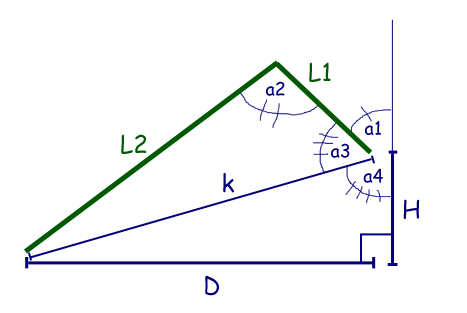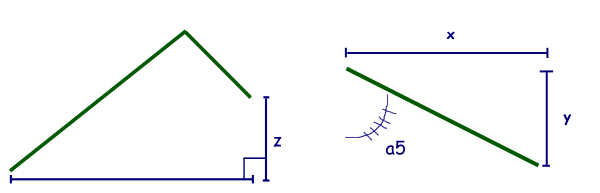## Sunday, February 14, 2010

### Spiderwheels: the third dimension and circles...

If you've been following this blog you'll know that I did most of the math needed for spider movement several months ago. However, to keep it simple I left out a dimension. Where once I only considered D and H I now need to consider x, y, and z.This weekend I pulled the equations back out in order to add the third dimension. Thankfully the previous equations could remain unchanged with the realization that:

D = sqrt(x2 + y2).
H = z.

Thus, I could still use these equations:

a1 = 180� - tan-1(D / H) - cos-1( (L12 - L22 + D2 + H2) / (2 * L1 * sqrt(D2 + H2)) ).

a2 = cos-1( (L12 + L22 - D2 - H2) / (2 * L1 * L2) ).

The third and final piece of this puzzle is an equation for the angle a5. Simple trigonometry gives us:

a5 = tan-1(x / y).

I've also been working on building out custom servo / leg libraries for my arduino. In order to prove that the equations for a1, a2, a5 work together fluidly and that my libraries are coming along I ran this expression:

```void loop(){
// circle
for(float i = 0; i<360; i = i +0.9){
goTo((70+(24*cos((i/180)*PI))), (0+(24*sin((i/180)*PI))), 30);
}
}
void goTo(float x, float y, float z){
int i;
for(i=leg1.findNeededPulses(x, y, z); i>0; i--){
leg1.pulseToPosition(x, y, z, i);
delay(10);
}
}
```

It simply draws a circle of radius 24mm at a height of 30mm. Here is a video of it in action: